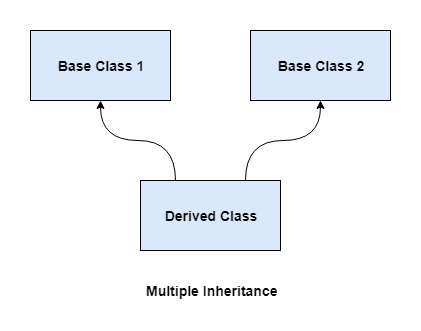# Multiple Inheritance in C++

Multiple inheritance occurs when a class inherits from more than one base class. So the class can inherit features from multiple base classes using multiple inheritance. This is an important feature of object oriented programming languages such as C++.

A diagram that demonstrates multiple inheritance is given below −A program to implement multiple inheritance in C++ is given as follows −

## Example

Live Demo

#include <iostream>
using namespace std;

class A {
public:
int a = 5;
A() {
cout << "Constructor for class A" << endl;
}
};
class B {
public:
int b = 10;
B() {
cout << "Constructor for class B" << endl;
}
};
class C: public A, public B {
public:
int c = 20;
C() {
cout << "Constructor for class C" << endl;
cout<<"Class C inherits from class A and class B" << endl;
}
};
int main() {
C obj;
cout<<"a = "<< obj.a <<endl;
cout<<"b = "<< obj.b <<endl;
cout<<"c = "<< obj.c <<endl;
return 0;
}

## Output

The output of the above program is given as follows −

Constructor for class A
Constructor for class B
Constructor for class C
Class C inherits from class A and class B
a = 5
b = 10
c = 20

In the above program, classes A and B are defined. This is given below −

class A {
public:
int a = 5;
A() {
cout << "Constructor for class A" << endl;
}
};
class B {
public:
int b = 10;
B() {
cout << "Constructor for class B" < endl;
}
};

Class C inherits from both classes A and B. It is an example of multiple inheritance. Class C definition is shown below −

class C: public A, public B {
public:
int c = 20;
C() {
cout << "Constructor for class C" << endl;
cout<<"Class C inherits from class A and class B" << endl;
}
};

In main() function, an object obj of class C is defined. The constructors of Class A, B and C are automatically called and their contents are displayed. Then the values of a, b and c are printed. These are data members of classes A, B and C respectively. The code snippet for this is as follows −

C obj;
cout<<"a = "<< obj.a <<endl;
cout<<"b = "<< obj.b <<endl;
cout<<"c = "<< obj.c <<endl;